DraftKings 2019 SEC Odds
+225
2.25 to 1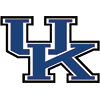Kentucky
23.2% implied probability

+375
3.75 to 1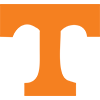Tennessee
15.9% implied probability

+475
4.75 to 1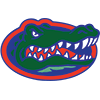Florida
13.1% implied probability

+700
7 to 1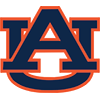Auburn
9.4% implied probability

+750
7.5 to 1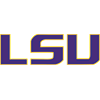Louisiana State
8.9% implied probability

+1200
12 to 1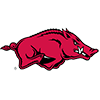Arkansas
5.8% implied probability

+1300
13 to 1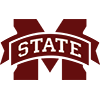Mississippi State
5.4% implied probability

+1500
15 to 1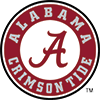Alabama
4.7% implied probability

+2000
20 to 1Georgia
3.6% implied probability

+2500
25 to 1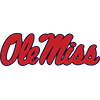Mississippi
2.9% implied probability

+2500
25 to 1Missouri
2.9% implied probability

+3500
35 to 1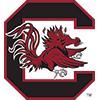South Carolina
2.1% implied probability

+6000
60 to 1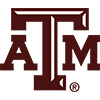Texas A&M
1.2% implied probability

+8000
80 to 1Vanderbilt
0.9% implied probability